# Parovichenko algebra

Parovičenko algebra

Let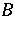be a Boolean algebra. If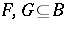, then one says thatprovided that for all finite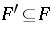and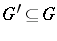one has. In addition,is said to be a Parovichenko algebra provided that it is both Cantor- and DuBois-Reymond-separable. This means that for all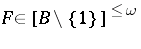and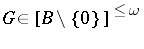such that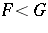there exists an elementsuch that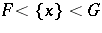.

The Parovichenko theorem from 1963 (see [a3]) asserts that under the continuum hypothesis (abbreviated CH), every Parovichenko algebra of size(i.e., of the cardinality of the continuum, cf. also Continuum, cardinality of the) is isomorphic to the Boolean algebra, where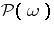is the power set algebra of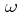andis its ideal of finite subsets. The theorem is proved by a transfinite induction withmany steps, where at each essential step of the process the separability properties of the Boolean algebras under consideration ensure that one can continue with the construction. This method goes back to W. Rudin [a7].

In 1978, E.K. van Douwen and J. van Mill proved the converse to Parovichenko's theorem in [a1]: If all Parovichenko algebras of size of the continuum are isomorphic, then the continuum hypothesis holds.

Parovichenko's theorem implies that every Boolean algebra of size at most that of the continuum can be embedded in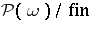under the continuum hypothesis. This result cannot be proved in ZFC alone (cf. also Set theory). In 1968, K. Kunen [a3] proved that in a model formed by adding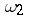Cohen reals to a model of the continuum hypothesis, there is nosequence of subsets ofwhich is strictly decreasing (modulo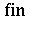). This implies that a Boolean algebra such as the clopen algebra of the ordinal space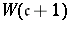cannot be embedded in. In 1978, E.K. van Douwen and T.C. Przymusiński [a2] used results of F. Rothberger [a6] to prove that there is a counterexample under the hypothesisThis is interesting, since it only involves a hypothesis on cardinal numbers.

Parovichenko's theorem has interesting consequences in topology. It implies, for example, that under the continuum hypothesis the Čech–Stone remainderof any zero-dimensional locally compact,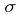-compact, non-compact spaceof weight at mostis homeomorphic to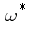, the Čech–Stone-remainder ofwith the discrete topology (cf. also Stone–Čech compactification; Topological structure (topology); Zero-dimensional space).